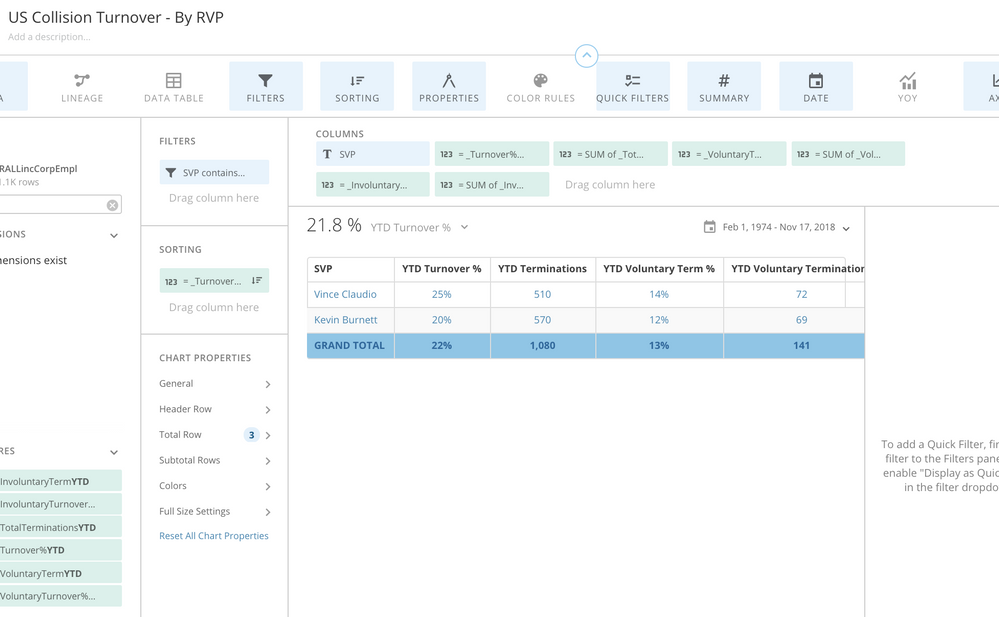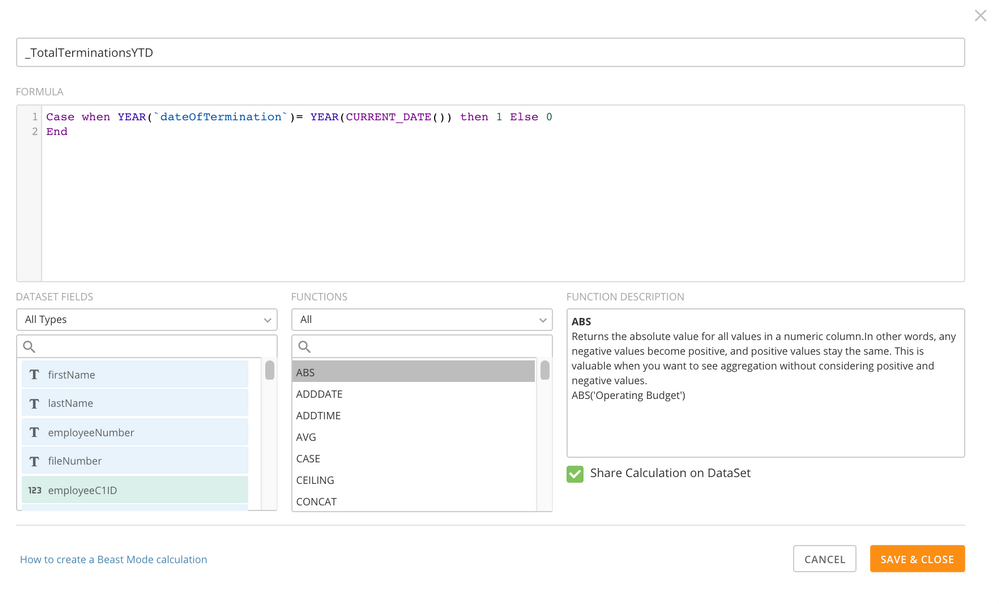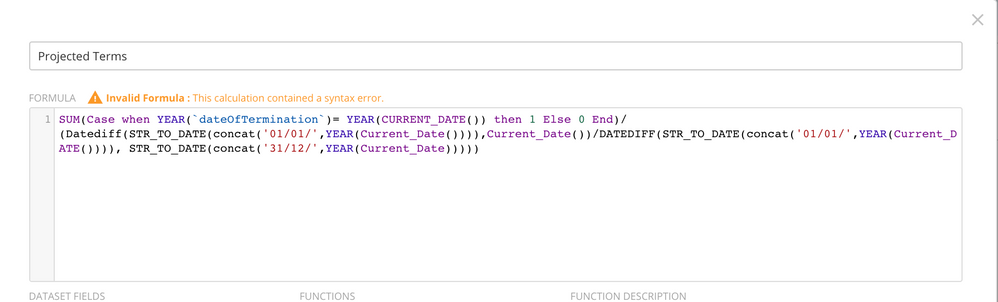# Annualized Terms and Turnover

Good Morning,

We have a turnover card that currently shows the YTD Turnover % along with the YTD terminations.

When calculating the annualized projected terms and turnover, I have used the YEARFRAC calculation in Excel. I'm not sure what I will need to do to recreate that column here in Domo.

Here's an example of the formula I would use in Excel to get the Annualized Projections:

Current Terms: 64

Today's Date: 5/17/18

Start of Year(1/1/18)

YEAR FRAC(Start of Year, Today's Date) {would return, .37777)

So to get the annualized terms I would take 64/.37777 {would return 169.411}

Current Terms/(YearFrac(StartofYear,TodaysDate,))

•

You can use beast mode:

`Current Terms/(Datediff(STR_TO_DATE(concat('01/01/',YEAR(Current_Date()))),Current_Date())/DATEDIFF(STR_TO_DATE(concat('01/01/',YEAR(Current_DATE()))), STR_TO_DATE(concat('31/12/',YEAR(Current_Date)))))`

Note: I assumed that Current Terms is a field in your dataset.

Let me know if it works or not,

• Hi Rahul,

Right now the YTD terms are being figured by using this beast mode in the data set:

Case when YEAR(`dateOfTermination`)= YEAR(CURRENT_DATE()) then 1 Else 0
End

What would be the best way to input that into the formula you gave me?

Thank you!

Becki

• Hey Becki,

I am not sure what you mean by YTD terms if its Current Terms then the formula could possibly be:

`SUM(Case when YEAR(`dateOfTermination`)= YEAR(CURRENT_DATE()) then 1 Else 0End)/(Datediff(STR_TO_DATE(concat('01/01/',YEAR(Current_Date()))),Current_Date())/DATEDIFF(STR_TO_DATE(concat('01/01/',YEAR(Current_DATE()))), STR_TO_DATE(concat('31/12/',YEAR(Current_Date)))))`

I am not sure if this will work or not. Please let me know,

• Hi there,

Hmm it didn't work. I'm not sure what would be helpful for you to see. Here's a snap shot of the top of the card, and the beast mode that's calculating the second column as a sum for the total YTD terms.